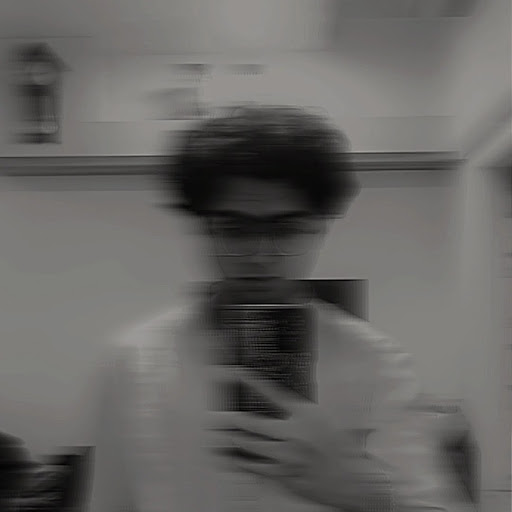Published

# Water Level Monitor With Oled Display Using Raspberry Pi

A Water level monitor with oled display display the percentage of bucket filled

BeginnerFull instructions provided1 hour334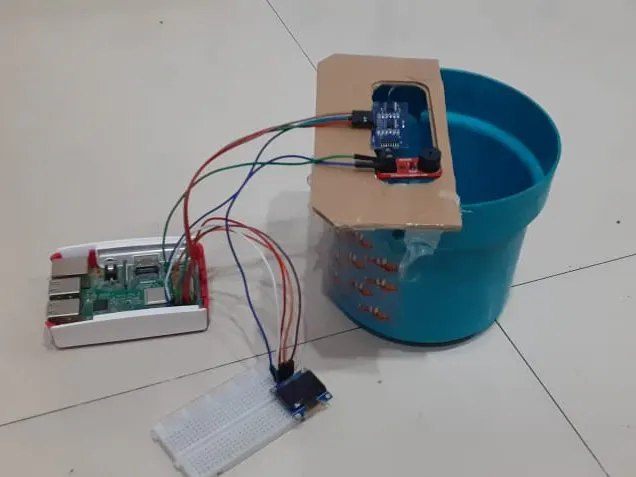## Things used in this project

### Hardware componentsRaspberry Pi 3 Model B Raspberry Pi
×1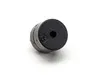Buzzer Buzzer
×1Ultrasonic Sensor - HC-SR04 (Generic) Ultrasonic Sensor
×1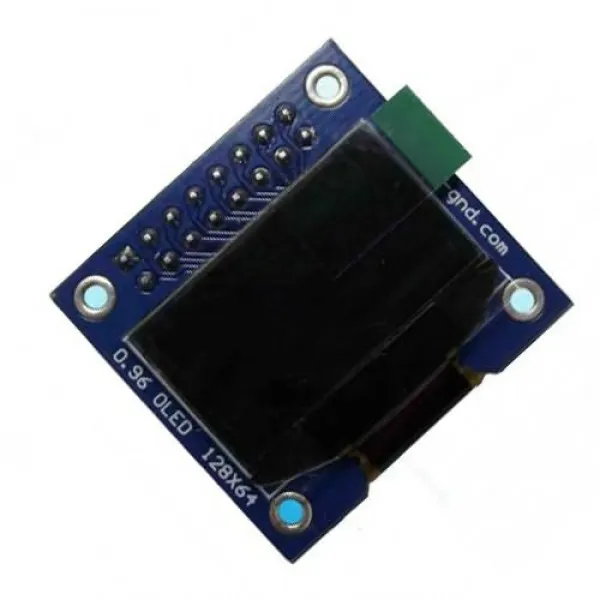ElectroPeak 0.96" OLED 64x128 Display Module Oled Display
×1Jumper wires (generic) Jumper Wires
×1

## Schematics

### Circuit Diagram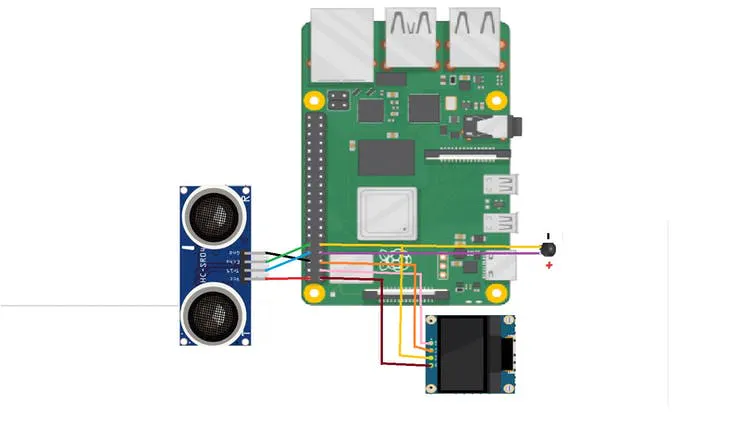## Code

### ultrasonic_water_level_with oled display.py

Python
Copy and paste it in Thonny Python Ide
```import time

from PIL import Image
from PIL import ImageDraw
from PIL import ImageFont
import RPi.GPIO as GPIO
import time

# Raspberry Pi pin configuration:
RST = 24
# Note the following are only used with SPI:
DC = 23
SPI_PORT = 0
SPI_DEVICE = 0

TRIG = 14
ECHO = 15
i=0

dist_from_base =  10 #Write the distance from the sensor to the base of the bucket
GPIO.setmode(GPIO.BCM)
GPIO.setup(TRIG ,GPIO.OUT)
GPIO.setup(ECHO,GPIO.IN)
GPIO.setup(4 ,GPIO.OUT)

# 128x32 display with hardware I2C:

# Initialize library.
disp.begin()

# Clear display.
disp.clear()
disp.display()

width = disp.width
height = disp.height
image = Image.new('1', (width, height))

draw = ImageDraw.Draw(image)

draw.rectangle((0,0,width,height), outline=0, fill=0)

shape_width = 20
# Move left to right keeping track of the current x position for drawing shapes.
# Draw an ellipse.

# Some other nice fonts to try: http://www.dafont.com/bitmap.php
#font = ImageFont.truetype('Minecraftia.ttf', 8)
GPIO.output(TRIG, False)
print("Starting.....")
time.sleep(2)

while True:

GPIO.output(TRIG, True)
time.sleep(0.00001)
GPIO.output(TRIG, False)

while GPIO.input(ECHO)==0:
pulse_start = time.time()

while GPIO.input(ECHO)==1:
pulse_stop = time.time()

pulse_time = pulse_stop - pulse_start

distance = pulse_time * 17150
distance = round(distance)
percentage = (distance * 100)/dist_from_base
percentage = 100 - percentage
percentage = round(percentage, 2)
percentage = str(percentage)
if distance > dist_from_base:
distance = dist_from_base
print(round(distance, 2));
distance = str(distance)
draw.text((x, top),    'Distance:',  font=font, fill=255)
draw.text((x+60, top), distance, font=font, fill=255)
draw.text((x+75, top), 'cm', font=font, fill=255)
draw.text((x, top+ 20), 'percentage of bucket filled:', font=font, fill=255)
draw.text((x, top+ 30), percentage, font=font, fill=255)
draw.text((x+30, top +30), '%', font=font, fill=255)

# Display image.
disp.image(image)
disp.display()
distance = float(distance)
time.sleep(1)
disp.clear()
draw.rectangle((0,0,width,height), outline=0, fill=0)

if distance < 4:
draw.text((x, top + 40),    'Water will overflow',  font=font, fill=255)
print("Water will overflow")
GPIO.output(4, True);
time.sleep(0.5)
GPIO.output(4, False);
time.sleep(0.5)
GPIO.output(4, True);
time.sleep(0.5)
GPIO.output(4, False);
time.sleep(0.5)
else:
GPIO.output(4, False);
# Write two lines of text.
```

## Credits

### shafin kothia

2 projects • 5 followers
I have been developing AIversity for robotics training and projects